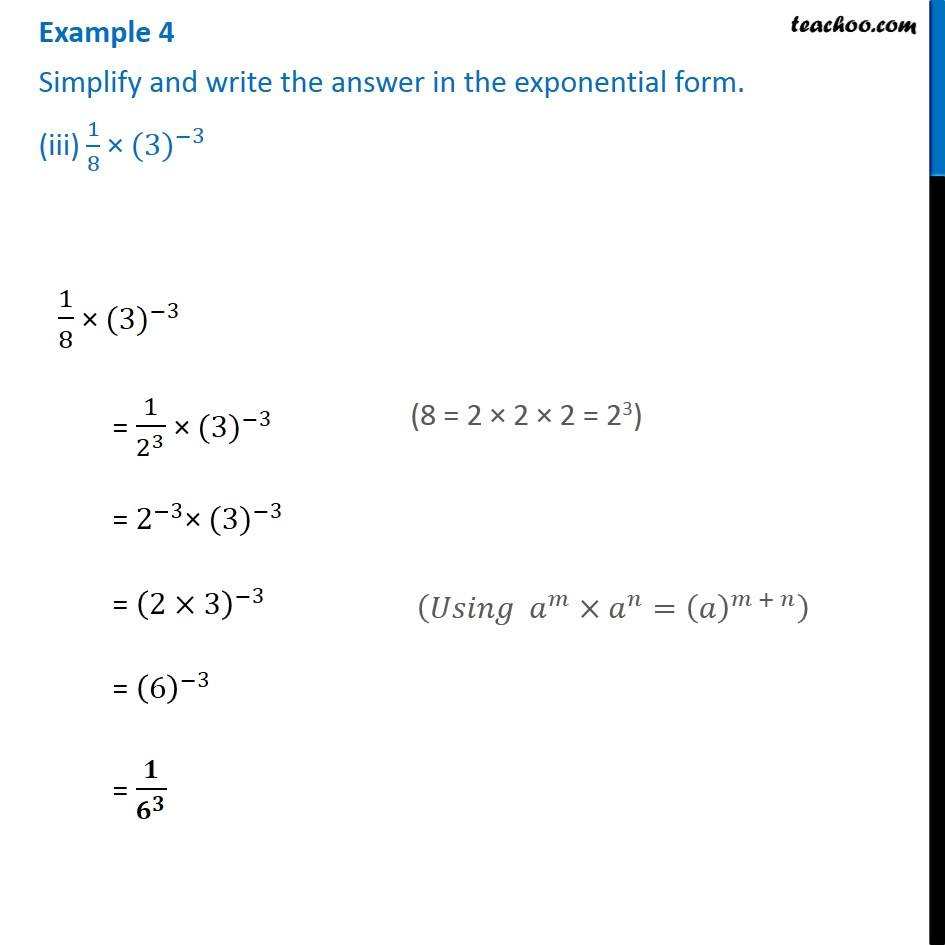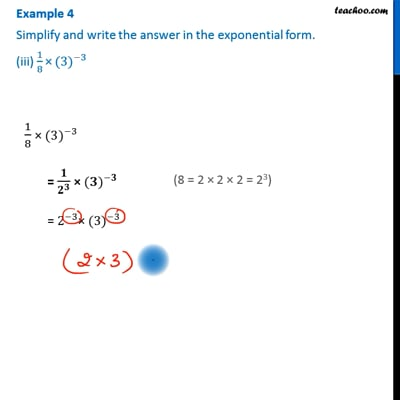Examples

Chapter 10 Class 8 Exponents and Powers
Serial order wiseThis video is only available for Teachoo black users

Learn in your speed, with individual attention - Teachoo Maths 1-on-1 Class

### Transcript

Example 4 Simplify and write the answer in the exponential form. (iii) 1/8 × 〖(3)〗^(−3) 1/8 × 〖(3)〗^(−3) = 1/2^3 × 〖(3)〗^(−3) = 2^(−3)× 〖(3)〗^(−3) = (2×3)^(−3) = (6)^(−3) = 𝟏/𝟔^𝟑 (8 = 2 × 2 × 2 = 23) (𝑈𝑠𝑖𝑛𝑔 𝑎^𝑚×𝑎^𝑛=(𝑎)^(𝑚 + 𝑛) )